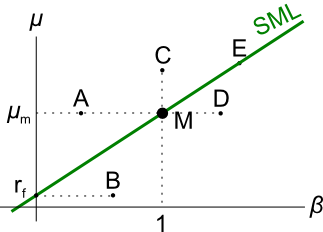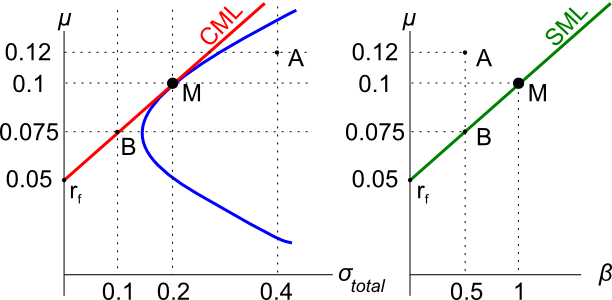# Fight Finance

#### CoursesTagsRandomAllRecentScores

Government bonds currently have a return of 5% pa. A stock has an expected return of 6% pa and the market return is 7% pa. What is the beta of the stock?

Government bonds currently have a return of 5%. A stock has a beta of 2 and the market return is 7%. What is the expected return of the stock?

Which statement(s) are correct?

(i) All stocks that plot on the Security Market Line (SML) are fairly priced.

(ii) All stocks that plot above the Security Market Line (SML) are overpriced.

(iii) All fairly priced stocks that plot on the Capital Market Line (CML) have zero idiosyncratic risk.

Select the most correct response:

The security market line (SML) shows the relationship between beta and expected return.

The security market line (SML) shows the relationship between beta and expected return.

Investment projects that plot on the SML would have:

Examine the following graph which shows stocks' betas $(\beta)$ and expected returns $(\mu)$:Assume that the CAPM holds and that future expectations of stocks' returns and betas are correctly measured. Which statement is NOT correct?Assets A, B, M and $r_f$ are shown on the graphs above. Asset M is the market portfolio and $r_f$ is the risk free yield on government bonds. Which of the below statements is NOT correct?Assets A, B, M and $r_f$ are shown on the graphs above. Asset M is the market portfolio and $r_f$ is the risk free yield on government bonds. Assume that investors can borrow and lend at the risk free rate. Which of the below statements is NOT correct?

Which of the following statements is NOT correct? Fairly-priced assets should:

Over-priced assets should NOT:

The market's expected total return is 10% pa and the risk free rate is 5% pa, both given as effective annual rates.

A stock has a beta of 0.7.

What do you think will be the stock's expected return over the next year, given as an effective annual rate?

The market's expected total return is 10% pa and the risk free rate is 5% pa, both given as effective annual rates.

A stock has a beta of 0.7.

In the last 5 minutes, bad economic news was released showing a higher chance of recession. Over this time the share market fell by 2%. The risk free rate was unchanged. What do you think was the stock's historical return over the last 5 minutes, given as an effective 5 minute rate?